Mapping method

method of images

A method in potential theory for solving certain boundary value problems for partial differential equations in a domain, where the fulfillment of the boundary conditions on the boundaryis achieved by the choice of additional field sources situated outsideand called image sources.

The greatest development of the mapping method occurred in electrostatics. Suppose, for example, that it is required to solve the Dirichlet problem for the Poisson equation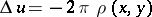in the half-plane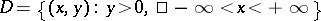with a given function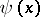on the boundary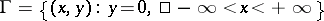, that is, it is required to find the potential of electrostatic charges of density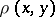situated in, with the condition that the potentialis fixed on. It is known that in order to solve this problem it suffices to know the Green functionrepresenting the potential of a unit point charge at the point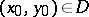when the boundaryis earthed, that is,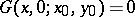. The solution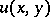of the original problem is expressed as follows in terms of: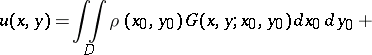(1)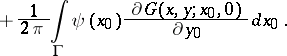When there is no boundary, the potential of a point charge can be written as a fundamental solutionof the Laplace equation. Adding a negative unit charge image at the point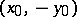and forming the sum of the potentials of these two charges, one obtains the desired Green function: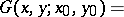(2)In the case of a strip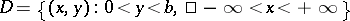, on reflecting a unit charge atwith respect to the lines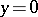and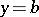one gets an infinite sequence of chargesat the points,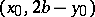,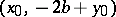,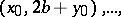respectively. The Green function in this case can be expressed in the form of an infinite series of potentials of point charges.

For a domainin the form of a disc,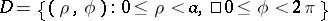, the image of a unit charge atis a negative unit charge at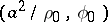, which is the image of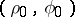under inversion relative to the circle.

Other configurations of boundaries are also possible, consisting of lines and circles, and the solution is obtained by constructing the corresponding sequence of charge images.

For the solution of the Dirichlet problem for the Poisson equation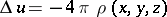in the half-spaceon reflecting the unit charge atwith respect to the plane, instead of (2) one obtains the formula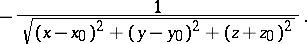In the case of a ball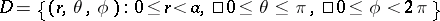it is necessary to apply the Kelvin transformation, and the image of the unit charge at the point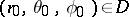is a charge of magnitude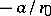at the point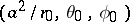, which is the image ofunder inversion with respect to the sphere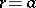. From this one obtains that if a solution of the Poisson equation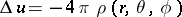is known in some domain, then the function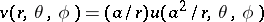gives a solution of the Poisson equation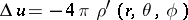with density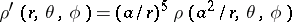in the domain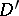that is the image ofunder inversion with respect to the sphere. In this form the mapping method is sometimes called the method of inversion. In applying the method of inversion it is necessary to pay attention to the fact that the boundary conditions are also transformed.

For more complicated domains in space, whose boundaries consist of some planes or spheres, it is also possible to apply infinite sequences of charge images. In conjunction with passage to limits, where one or more sources go to infinity, the mapping method makes it possible to solve complicated problems, such as the determination of the potential of an electrostatic field in the case of a conducting ball placed in a field that is homogeneous at infinity.

In the case of the Helmholtz equation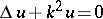, the mapping method is applicable only for domains bounded by lines or for domains in space bounded by planes and uses the corresponding fundamental solutions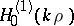,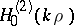or,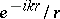.